• 对灰度图像进行二值化处理，小于某个阈值设为0，即黑色，大于阈值的设为1，即白色。
• matlab对图像二值化处理，matlab对图像二值化处理，matlab对图像二值化处理matlab
• 初学图像处理的人，一般首先熟悉图像格式，图像存储方式，8位灰度图，24位彩色图等基础知识，然后接触到的图像算法一般都是图像直方图、图像二值化处理等基础算法。二值化算法作为图像处理入门级算法，在很多场合都...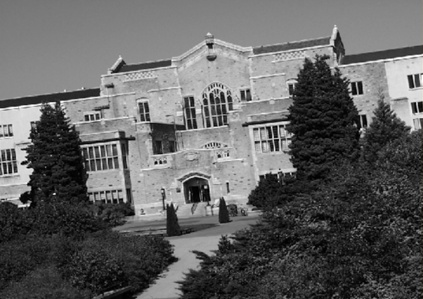初学图像处理的人，一般首先熟悉图像格式，图像存储方式，8位灰度图，24位彩色图等基础知识，然后接触到的图像算法一般都是图像直方图、图像二值化处理等基础算法。二值化算法作为图像处理入门级算法，在很多场合都有应用。常用的二值化算法是固定阈值二值化，算法本身很简单，机器视觉处理的很多都是8位灰度图像，灰度值从0到255,。所谓二值化就是给定一个阈值，让小于这个阈值的灰度值为0，大于等于这个阈值的设为255，这样在图像上显示出来就是一幅黑白图像。当然，很多资料介绍的是小于阈值为0，大于阈值为1。这其实是一个意思。但是，如果想看到显示效果，一般不会用1来表示亮的点，而是用255。如果熟悉了最早我写的opencv对于mat数据的操作，要实现这种固定阈值算法是非常简单的。但是，opencv已经写好了二值化算法，也不需要我们自己写了。下面看一下具体的例子，代码在vs2015+opencv4.0.1上实现。如果已经配置好了opencv，可以直接运行。#include "iostream"#include "opencv2opencv.hpp"using namespace std;int main(int argc, char ** argv){ Mat src = imread("e:img1.ppm", 0); Mat dst; threshold(src, dst, 100, 255, 0); Mat adpDst; adaptiveThreshold(src, adpDst, 100, 0, 0, 31,-20); namedWindow("src", 0); imshow("src", src); namedWindow("dst", 0); imshow("dst", dst); namedWindow("adp", 0); imshow("adp", adpDst); waitKey(0); return 0;}可以看到，算法本身很简单，只需要一个函数threshold就可以实现。第一个参数是输入图像，第二个是输出图像，第三个是阈值，第四个是指将大于等于阈值的灰度值设置为多大的值，最后一个参数是二值化方式。在opencv里面用了一个枚举类型来定义。如下：enum ThresholdTypes {THRESH_BINARY = 0, THRESH_BINARY_INV = 1, THRESH_TRUNC = 2,THRESH_TOZERO = 3, THRESH_TOZERO_INV = 4, THRESH_MASK = 7,THRESH_OTSU = 8, THRESH_TRIANGLE = 16 };在这几种二值化方式的中，最常用的也就是前面两种。有的人还喜欢用THRESH_OTSU进行二值化，这是大律法。然而，如果直接用大律法进行二值化，实际效果并不是很好，因为这是全局阈值，比较好的二值化方法应该用局部阈值，毕竟图像上每个地方的灰度值差别是比较大的。所以，opencv里面有提供了一个局部自适应二值化函数adaptiveThreshold。这个函数的效果比较好，但是，很多人用这个函数的时候，却不知道参数怎么设置比较好，结果往往出来的效果不理想，反而认为算法不好。其实，就算固定阈值二值化算法，在很多时候都能得到很好的效果，只不过我们很多人不是很清楚算法怎么配合使用而已。比如，我们设置参数的时候，对于adaptiveThreshold算法，设置的块大小很多人都是3、5、7。好像觉得把块设大一点图像就不对了一样，对于adaptiveThreshold的最后一个参数，其实还可以设置为负值，可能最后得到的效果就完全不一样。所以，这就是我们对算法不够了解，只知道如何调用这些函数，却不知道怎么使用是最好的。另外，对于threshold函数，由于只有一个固定阈值，而很多时候我们可能需要分段阈值，有可能图像上的感兴趣区域灰度值在100到180之间，那么这个函数就需要我们自己改造一下才能够达到效果。此外，其实很多时候是不直接对原图进行二值化操作的，这种效果并不好，这也是很多人觉得threshold函数效果不好的原因，给个提示，如果你先将图像进行一下边缘检测，再用这个函数试试，可能效果就完全不一样了，当然边缘检测的函数也要有选择，不能随便哪个函数都可以。上面代码只是展示了threshold和adaptiveThreshold两个函数的调用方法，具体的参数怎么调节才能到达最好的效果，还需要根据具体的图像来确定，在视觉检测中，一般要将参数发布出来，供技术人员调试，不然很难达到实际检测需求。下面的图是上面两种算法的结果，可以参考一下。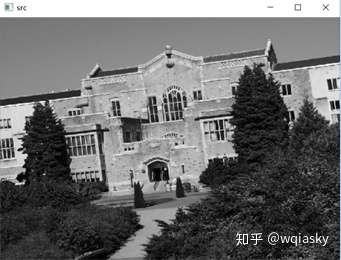图1 原图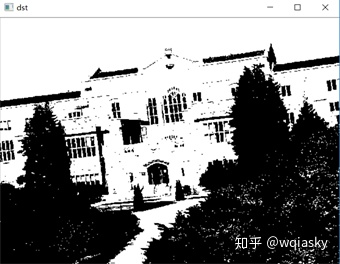图2 固定阈值二值化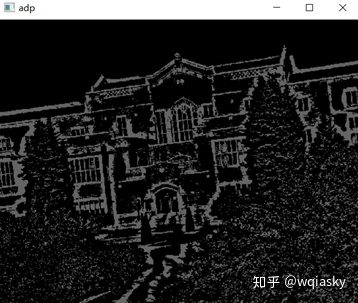图3 自适应二值化
展开全文• 这是一款实现图像的灰度二值化处理功能源码，该源码主要是利用opencv2.framework进行图像的灰度二值化处理，可以对图像的某个特定矩形区域进行二值化处理，效果如图所示，上方图片是原图，下方是对上方的图片的某个...IOS源码
• 图片灰度和二值化处理
图片灰度和二值化处理<?php function adaptiveThreshold($file){if(!$ex = getimagesize($file)){return false;}// 打开图片switch($ex){case IMAGETYPE_JPEG:case IMAGETYPE_JPEG2000:if(!$im = imageCreateFromJpeg($file)){return false;}break;case IMAGETYPE_PNG:$im = imageCreateFromPng($file);break;case IMAGETYPE_GIF:$im = imageCreateFromGif($file);break;case IMAGETYPE_BMP:$im = imageCreateFromBmp($file);break;default :return false;}$gray = array_fill(0,$ex,array_fill(0, $ex, 0));// 转为灰阶图像foreach($gray as $y => &$row){foreach($row as$x => &$Y){$rgb = imagecolorat($im,$x, $y);// 根据颜色求亮度$B = $rgb & 255;$G = ($rgb >> 8) & 255;$R = ($rgb >> 16) & 255;$Y = ($R * 19595 +$G * 38469 + $B * 7472) >> 16;}}unset($row, $Y);// 自动求域值$back = 127;do{$crux =$back;$s =$b = $l =$I = 0;foreach($gray as$row){foreach($row as$Y){if($Y <$crux){$s +=$Y;$l++;}else{$b += $Y;$I++;}}}$s =$l ? floor($s /$l) : 0;$b =$I ? floor($b /$I) : 0;$back = ($s + $b) >> 1;}while($crux != $back);// 二值化$bin = $gray;foreach($bin as &$row){foreach($row as &$Y){$Y = $Y <$crux ? 0 : 1;}}return array($gray,$bin,);}if(empty($_GET["img"])){exit("Please use$_SERVER[SCRIPT_NAME]?img=local/image/path");}if(!$im = adaptiveThreshold($_GET["img"])){exit("error!");}$img = imagecreate(count($im), count($im) * 2);$rgb = array(imagecolorallocate($img, 0, 0, 0),imagecolorallocate($img, 255, 255, 255),);$x =$y = 0;$colors = array(0 =>$rgb,255 => $rgb);foreach($im as $row){do{if(isset($colors[$row[$x]])){$c =$colors[$row[$x]];}else{$c =$colors[$row[$x]] = imagecolorallocate($img,$row[$x],$row[$x],$row[$x]);}// imagesetpixel($img, $x,$y, $rgb[$row[$x] < 128 ? 0 : 1]);imagesetpixel($img, $x,$y, $c);}while(isset($row[++$x]));$x = 0;$y++;}foreach($im as $row){do{imagesetpixel($img, $x,$y, $rgb[$row[$x]]);}while(isset($row[++$x]));$x = 0;$y++;}header("Content-Type: image/gif");imagegif($img);以上就是图片灰度和二值化处理的内容，更多相关内容请关注PHP中文网(www.php.cn)！本文原创发布php中文网，转载请注明出处，感谢您的尊重！
展开全文• 用于图像二值化处理的简单代码。可以自动确定合适的阈值，并显示处理前、处理后的效果图。matlab
•matlab
• Python语言是一门比较简单好用的语言，其图像处理也是比较重要的一种应用，那么该怎么实现对对一个图片的二值化处理呢，下面小编给出具体的方法。1、打开Python的shell界面，如图所示。载入相关的库包，代码如下：...Python语言是一门比较简单好用的语言，其图像处理也是比较重要的一种应用，那么该怎么实现对对一个图片的二值化处理呢，下面小编给出具体的方法。1、打开Python的shell界面，如图所示。载入相关的库包，代码如下：from skimage import io,data,color读取图片，代码如下：img=data.注，这里读取的是工具包中的图片，大家也可以读取自己的图片，可参考实例“如何采用Python读取一个图像”，具体代码如下：img_name="D:\\WinPython-64bit-3.5.3.0Qt5\\notebooks\\hashiqi.jpg"img=io.imread(img_name,as_grey=False)将图片进行灰度化处理，具体指令如下：img_gray=color.rgb2gray(img)对图片进行二值化处理，代码如下；rows,cols=img_gray.shapefor i in range(rows):for j in range(cols):if (img_gray[i,j]<=0.5):img_gray[i,j]=0else:img_gray[i,j]=1查看结果的代码：io.imshow(img_gray)io.show查看我们的效果如下图。前图是二值化之前，后图是二值化之后。更多Python相关技术文章，请访问Python教程栏目进行学习！
展开全文• 在指纹识别算法中，由于现有指纹采集设备的不完善性，均需对采集的图像进行二值化处理，这个过程是相对难突破的一个不可或缺的重要环节。二值化是将含有噪声的灰度图像处理成适于特征提取的二值图像，其处理结果的...
• 提出了一种指纹图像二值化处理方法，该方法利用维纳滤波对原始图像进行预处理，然后采用连续块方向图计算指纹方向，结合方向信息用方向滤波模板对图像进行增强二值化。实验结果表明，该算法可以消除孔洞、去除粘线、...
• 彩色图像的二值化处理彩色图像的二值化处理彩色图像的二值化处理彩色图像的二值化处理彩色图像的二值化处理
• 图像处理 二值化处理 滚球系统单点定位 把程序直接烧录探索者可直接测试
• c#图像处理之图形灰度化二值化处理。通过读取图像，实现图像灰度化，二值化功能，并保存。
• ## opencv 二值化处理

千次阅读 2017-08-29 15:41:33
二值化处理图像的最大缺点在于对于光照特别敏感 一 二值化处理函数double threshold( InputArray src, OutputArray dst, double thresh, double maxval, int type ); 第一个参数是输入图像 第二个参数是输出图像...
二值化处理图像的最大缺点在于对于光照特别敏感
一  二值化处理函数double threshold( InputArray src, OutputArray dst, double thresh, double maxval, int type );
第一个参数是输入图像
第二个参数是输出图像
第三个参数是设定的阈值
第四个参数是最大阈值
第五个是函数类型
--------其中函数类型有
CV_THRESH_BINARY, //表示如果当前像素点的灰度值大于阈值则将输出图像的对应位置像素值置为255，否则为0
CV_THRESH_BINARY_INV,//如果当前像素点灰度值大于阈值则将像素值置为0，否则为255
CV_THRESH_TRUNC, //如果像素值大于阈值则将阈值赋给当前像素，否则保证当前像素值不变
CV_THRESH_TOZERO,//如果当前灰度值大于阈值则用阈值代替当前灰度值，否则将灰度值置0
CV_THRESH_TOZERO_INV,//与上面相反，如果大于则为0，小于则为阈值

二：自适应阈值二值化//其阈值会跟随像素值不同而改变
第三个参数是阈值最大值
第五个参数是像素取值类型与上面的一样
第六个是领域范围（内核大小），其只能取大于1的奇数
第七个是加权平均常数，最后通过高斯或中值滤波求得的像素值再减去这个加权平均后才是阈值

效果图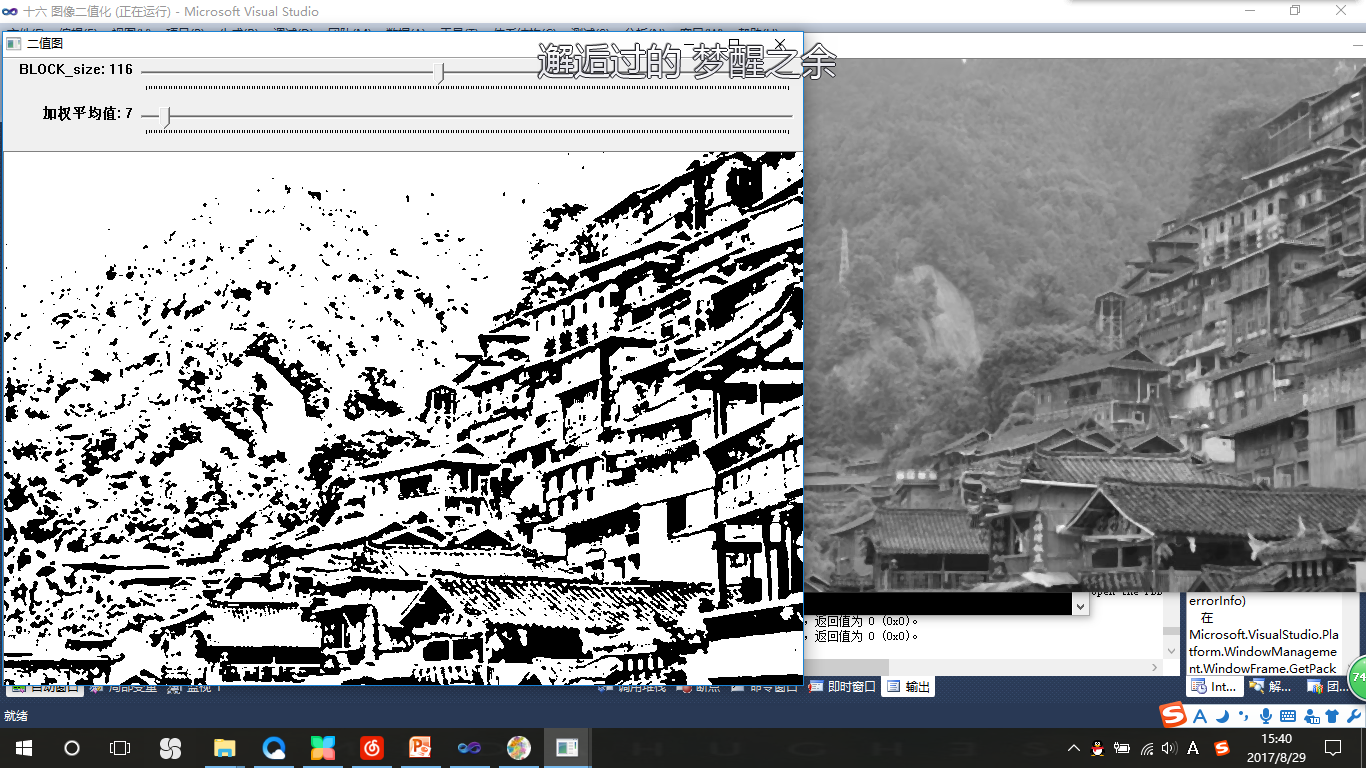展开全文• #include "stdafx.h"//对一张图片进行二值化处理 IplImage *pSrclmg =NULL;//载入的图片IplImage *pDeclmg =NULL;//生成的图像 void onTrackerSlid(int thresth){ /*int i,j,k; //设置一个阈值，如果大于这个阈值...
• 图像二值化处理时图像处理过程中非常常见的一种操作，在python中比较常用的主要是opencv,pil两个第三库，对比来看的话，opencv使用要更加的方便灵活， 文本主要介绍以下基于opencv的图像二值化处理方法。 首相我们...opencv python 计算机视觉
• 提出了一种新的带阻滤波二值化处理方法。首先，用带阻滤波和高斯低通滤波算法分别处理原始图像；然后，在高斯低通滤波图像中，用区域增长方法提取指纹的轮廓；最后，用轮廓线对带阻滤波指纹图像进行背景填充，把二值...
• 主要介绍了python 图片二值化处理（处理后为纯黑白的图片），文中通过示例代码介绍的非常详细，对大家的学习或者工作具有一定的参考学习价值，需要的朋友们下面随着小编来一起学习学习吧...